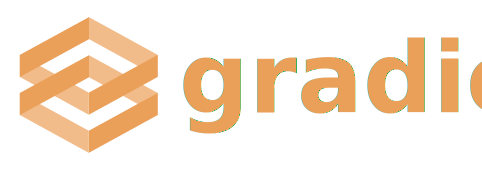## Machine Learning Examples

After you're familiar with the basics of Gradio library, you'll probably want to try it on a machine learning model. Let's see Gradio working with a few machine learning examples.

### Image Classification in Tensorflow / KerasWe'll start with the Inception Net image classifier, which we'll load using Tensorflow! Since this is an image classification model, we will use the `Image` input interface. We'll output a dictionary of labels and their corresponding confidence scores with the `Label` output interface. (The original Inception Net architecture can be found here)

``````import gradio as gr
import tensorflow as tf
import numpy as np
import requests

inception_net = tf.keras.applications.InceptionV3() # load the model

response = requests.get("https://git.io/JJkYN")
labels = response.text.split("\n")

def classify_image(inp):
inp = inp.reshape((-1, 299, 299, 3))
inp = tf.keras.applications.inception_v3.preprocess_input(inp)
prediction = inception_net.predict(inp).flatten()
return {labels[i]: float(prediction[i]) for i in range(1000)}

image = gr.inputs.Image(shape=(299, 299))
label = gr.outputs.Label(num_top_classes=3)

gr.Interface(fn=classify_image, inputs=image, outputs=label, capture_session=True).launch()``````

This code will produce the interface below. The interface gives you a way to test Inception Net by dragging and dropping images, and also allows you to use naturally modify the input image using image editing tools that appear when you click EDIT. Notice here we provided actual `gradio.inputs` and `gradio.outputs` objects to the Interface function instead of using string shortcuts. This lets us use built-in preprocessing (e.g. image resizing) and postprocessing (e.g. choosing the number of labels to display) provided by these interfaces. Finally, we use `capture_session=True` to ensure compatibility with TF 1.x. Try it out in your device or run it in a colab notebook!The above code also shows how you can add interpretation to your interface. You can use our out of the box functions for text and image interpretation or use your own interpretation functions. To use the out of the box functions just specify “default” for the interpretation parameter (Note: this only works for text/image input and label outputs).

``````gr.Interface(classify_image, image,
label, capture_session=True, interpretation="default").launch();
``````

### Image Classification in PytorchLet's now wrap a very similar model, ResNet, except this time in Pytorch. We'll also use the `Image` to `Label` interface. (The original ResNet architecture can be found here)

``````import gradio as gr
import torch
from torchvision import transforms
import requests
from PIL import Image

response = requests.get("https://git.io/JJkYN")
labels = response.text.split("\n")

def predict(inp):
inp = Image.fromarray(inp.astype('uint8'), 'RGB')
inp = transforms.ToTensor()(inp).unsqueeze(0)
prediction = torch.nn.functional.softmax(model(inp), dim=0)
return {labels[i]: float(prediction[i]) for i in range(1000)}

inputs = gr.inputs.Image()
outputs = gr.outputs.Label(num_top_classes=3)
gr.Interface(fn=predict, inputs=inputs, outputs=outputs).launch()``````

This code will produce the interface below.### Text Generation with Transformers (GPT-2)Let's wrap a `Text` to `Text` interface around GPT-2, a text generation model that works on provided starter text. Learn more about GPT-2 and similar language models.

``````import gradio as gr
import tensorflow as tf

tokenizer = GPT2Tokenizer.from_pretrained("gpt2")

def generate_text(inp):
input_ids = tokenizer.encode(inp, return_tensors='tf')
beam_output = model.generate(input_ids, max_length=100, num_beams=5,
no_repeat_ngram_size=2, early_stopping=True)
output = tokenizer.decode(beam_output, skip_special_tokens=True, clean_up_tokenization_spaces=True)
return ".".join(output.split(".")[:-1]) + "."

output_text = gr.outputs.Textbox()
gr.Interface(generate_text,"textbox", output_text).launch()``````

This code will produce the interface below. That's all that's needed!### Answering Questions with BERT-QAWhat if our model takes more than one input? Let's wrap a 2-input to 1-output interface around BERT-QA, a model that can answer general questions. As shown in the code, Gradio can wrap functions with multiple inputs or outputs, simply by taking the list of components needed. The number of input components should match the number of parameters taken by `fn`. The number of output components should match the number of values returned by `fn`. Similarly, if a model returns multiple outputs, you can pass in a list of output interfaces.

``````import gradio as gr
from bert import QA

def qa_func(context, question):

gr.Interface(qa_func,
[
gr.inputs.Textbox(lines=7, label="Context"),
gr.inputs.Textbox(label="Question"),
],

This code will produce the interface below.### Numerical Interfaces: Titanic Survival ModelMany models have numeric or categorical inputs, which we support with a variety of interfaces. Let's wrap multiple input to label interface around a Titanic survival model. We've hidden some of model-related code below, but you can see the full code on colab.

``````import gradio as gr
import pandas as pd
import numpy as np
import sklearn

def predict_survival(sex, age, fare):
df = pd.DataFrame.from_dict({'Sex': [sex], 'Age': [age], 'Fare': [fare]})
df = encode_sex(df)
df = encode_fares(df)
df = encode_ages(df)
pred = clf.predict_proba(df)
return {'Perishes': pred, 'Survives': pred}

age = gr.inputs.Slider(minimum=0, maximum=120, default=22, label="Age")
fare = gr.inputs.Slider(minimum=0, maximum=1000, default=100, label="Fare (british pounds)")

gr.Interface(predict_survival, [sex, age, fare], "label", live=True).launch()``````

This code will produce the interface below.### Multiple Image Classifiers: Comparing Inception to MobileNetWhat if we want to compare two models? The code below generates a UI that takes one image input and returns predictions from both Inception and MobileNet. We'll also add a title, description, and sample images.

``````import gradio as gr
import tensorflow as tf
import numpy as np
from PIL import Image
import requests
from urllib.request import urlretrieve

response = requests.get("https://git.io/JJkYN")
labels = response.text.split("\n")

urlretrieve("https://www.sciencemag.org/sites/default/files/styles/article_main_large/public/cc_BE6RJF_16x9.jpg?itok=nP17Fm9H","monkey.jpg")
urlretrieve("https://external-preview.redd.it/lG5mI_9Co1obw2TiY0e-oChlXfEQY3tsRaIjpYjERqs.jpg?auto=webp&s=ea81982f44b83efbb803c8cff8953ee547624f70","bicycle.jpg")

mobile_net = tf.keras.applications.MobileNetV2()
inception_net = tf.keras.applications.InceptionV3()

def classify_image_with_mobile_net(im):
im = Image.fromarray(im.astype('uint8'), 'RGB')
im = im.resize((224, 224))
arr = np.array(im).reshape((-1, 224, 224, 3))
arr = tf.keras.applications.mobilenet.preprocess_input(arr)
prediction = mobile_net.predict(arr).flatten()
return {labels[i]: float(prediction[i]) for i in range(1000)}

def classify_image_with_inception_net(im):
# Resize the image to
im = Image.fromarray(im.astype('uint8'), 'RGB')
im = im.resize((299, 299))
arr = np.array(im).reshape((-1, 299, 299, 3))
arr = tf.keras.applications.inception_v3.preprocess_input(arr)
prediction = inception_net.predict(arr).flatten()
return {labels[i]: float(prediction[i]) for i in range(1000)}

imagein = gr.inputs.Image()
label = gr.outputs.Label(num_top_classes=3)
sample_images = [
["monkey.jpg"],
["sailboat.jpg"],
["bicycle.jpg"]
]
gr.Interface(
[classify_image_with_mobile_net, classify_image_with_inception_net],
imagein,
label,
title="MobileNet vs. InceptionNet",
description="Let's compare 2 state-of-the-art machine learning models
that classify images into one of 1,000 categories: MobileNet (top),
a lightweight model that has an accuracy of 0.704, vs. InceptionNet
(bottom), a much heavier model that has an accuracy of 0.779.",
examples=sample_images).launch();``````

This code will produce the interface below. Looks like MobileNet is about 3 times faster!